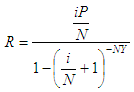# Calculate Monthly Loan Payments

At the bottom you can find an online spreadsheet to calculate monthly loan payments.

The spreadsheet calculates the amount required as regular payments in order to repay a loan over a specified time period. You must enter the principal, the interest rate charged, the number of payments to be made per year and the number of years to pay. This method assumes all installment payments are equal.

This is a simple loan payment calculator based on this formula:where

R = regular payment
P = principal
i = annual interest rate
N = number of payments per year
Y = number of years

### Example:

You can use this as a home loan monthly payment calculator... Let's say that you want to know what you must pay on a loan of \$400,000 at 8% if payments are do be made monthly for 10 years.

Just fill in the blanks of the calculator...

Principal = 400,000.00
Annual Interest Rate = 8%
Nr. of Payments / yr = 12
Term in years = 10

And the calculator delivers...

Regular Payments = 4,853.10

 You have to allow JavaScript codes on your navigator, otherwise the calculator won't work.

 Enter the needed values... (do not use commas) Principal: Interest Rate/year: % Number of Payments/year: Term in years: Regular Payments:

From 'Calculate Monthly Loan Payments' to home

From 'Calculate Monthly Loan Payments' to Free Online Calculators